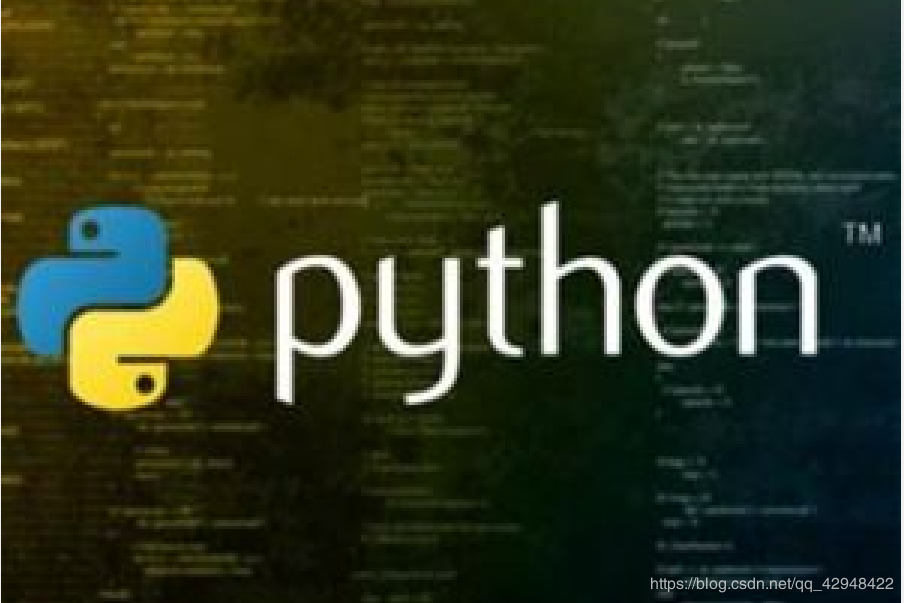# Python递归函数如何写？正确的Python递归函数用法！

02/01 14:00fact(n) = n! = 1 x 2 x 3 x … x (n-1) x n = (n-1)! x n = fact(n-1) x n

ps:另外很多人在学习Python的过程中，往往因为没有好的教程或者没人指导从而导致自己容易放弃，为此我建了个Python交流.裙 ：一久武其而而流一思（数字的谐音）转换下可以找到了，里面有最新Python教程项目可拿,不懂的问题多跟里面的人交流，都会解决哦！

def fact(n):
if n==1:
return 1
return n * fact(n - 1)

fact(1)
1

fact(5)
120

fact(100)
93326215443944152681699238856266700490715968264381621468592963895217599993229915608941463976156518286253697920827223758251185210916864000000000000000000000000

===> fact(5)
===> 5 * fact(4)
===> 5 * (4 * fact(3))
===> 5 * (4 * (3 * fact(2)))
===> 5 * (4 * (3 * (2 * fact(1))))
===> 5 * (4 * (3 * (2 * 1)))
===> 5 * (4 * (3 * 2))
===> 5 * (4 * 6)
===> 5 * 24
===> 120

fact(1000)
Traceback (most recent call last):
File “”, line 1, in
File “”, line 4, in fact

File “”, line 4, in fact
RuntimeError: maximum recursion depth exceeded in comparison

def fact(n):
return fact_iter(n, 1)

def fact_iter(num, product):
if num == 1:
return product
return fact_iter(num - 1, num * product)

fact(5)对应的fact_iter(5, 1)的调用如下：

===> fact_iter(5, 1)
===> fact_iter(4, 5)
===> fact_iter(3, 20)
===> fact_iter(2, 60)
===> fact_iter(1, 120)
===> 120

Python标准的解释器没有针对尾递归做优化，任何递归函数都存在栈溢出的问题。# -- coding: utf-8 --

def move(n, a, b, c):

``````if n == 1:
print(a, '-->', c)
``````

# A --> C

move(3, ‘A’, ‘B’, ‘C’)
Run

0
0 收藏

### 作者的其它热门文章0 评论
0 收藏
0